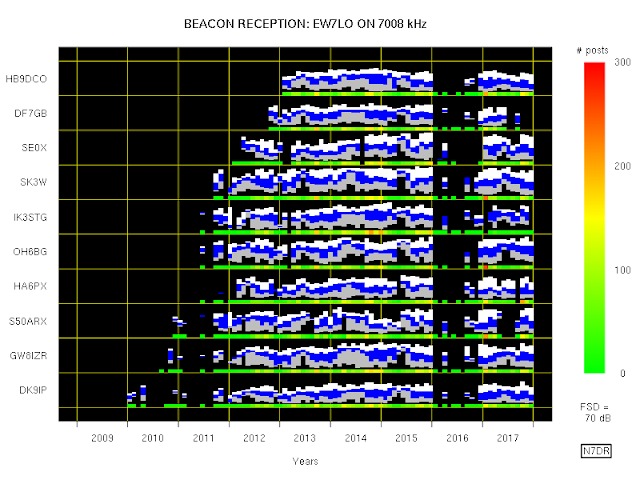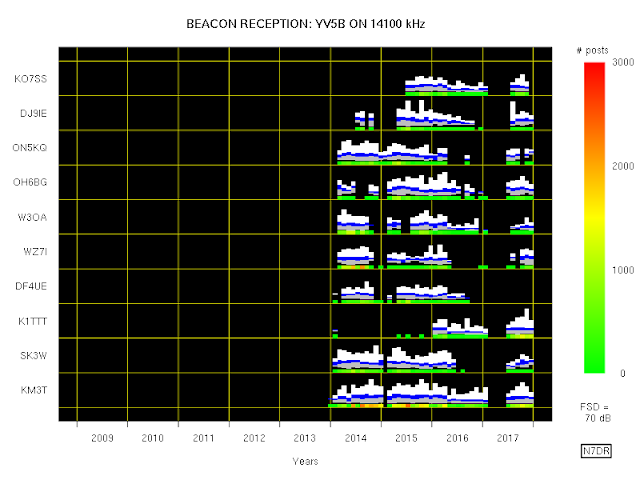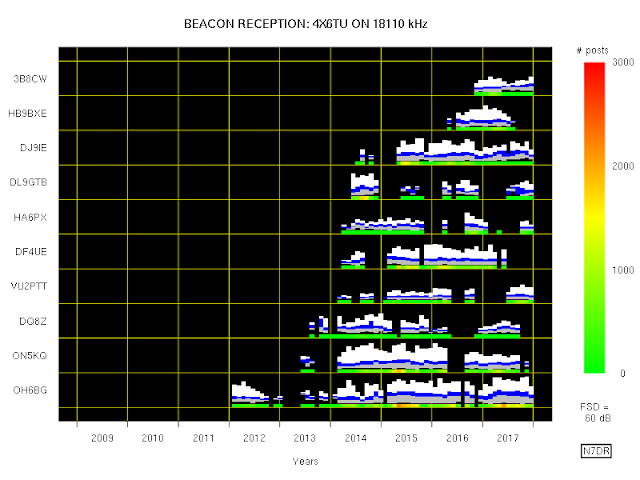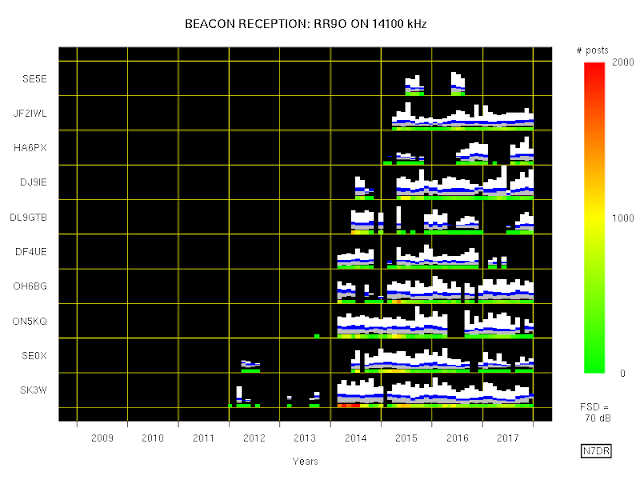## 2019-01-08

### Long-Term RBN Reception of HF Beacons, 2009 to 2017

This post brings this one up to the end of 2017, based on the beacon stations listed here.

Below are figures similar to the ones in that post. Here is the description copied from that post, with minor emendations as necessary (mostly to allow for a slight change in the way that the data are displayed):
1. The ordinate for each of the strip charts ranges between 0 dB and the value shown as FSD (i.e., full scale deflection) near the bottom right-hand corner; in this case, the maximum value of each strip is therefore 80 dB.
2. The value plotted in this manner is the value denoted SNR by the RBN.  Remember that the RBN has an odd definition of SNR.
3. The abscissa is divided into a number of bins of equal duration. On each plot there are 100 such bins; because the duration covered by each plot is nine years, each bin therefore covers a little less than one month.
4. At the bottom of each strip chart is a coloured bar. Each bin is coloured so as to represent the total number of times that the RBN station spotted the beacon in the period covered by the bin. Thus, in the first plot below,  the short red bar towards the bottom right-hand corner indicates that WZ7I spotted the 7026 kHz I1MMR beacon about 600 times in the course of a period slightly longer than one month.
5. For the period covered by each bin, the lower quartile of SNR readings is coloured grey, the upper quartile is coloured white, and the middle two quartiles are coloured blue..
6. The vertical order of the various RBN stations is determined solely by the chronological order in which each station first spotted the beacon.